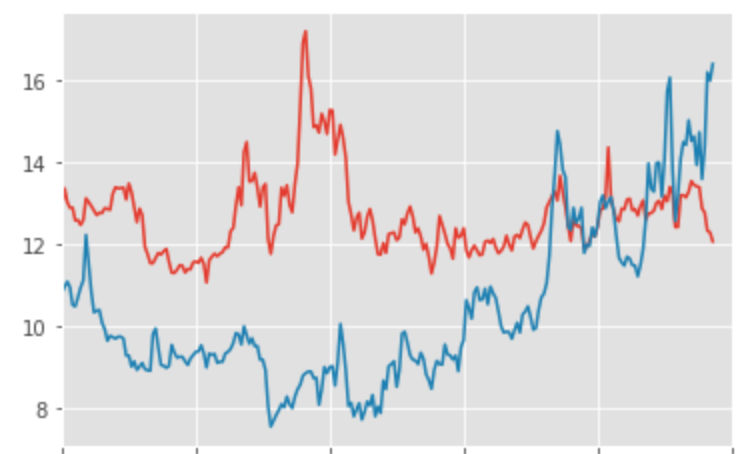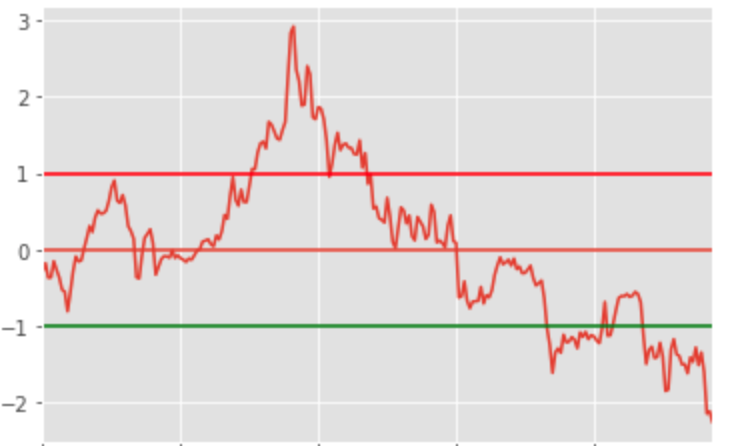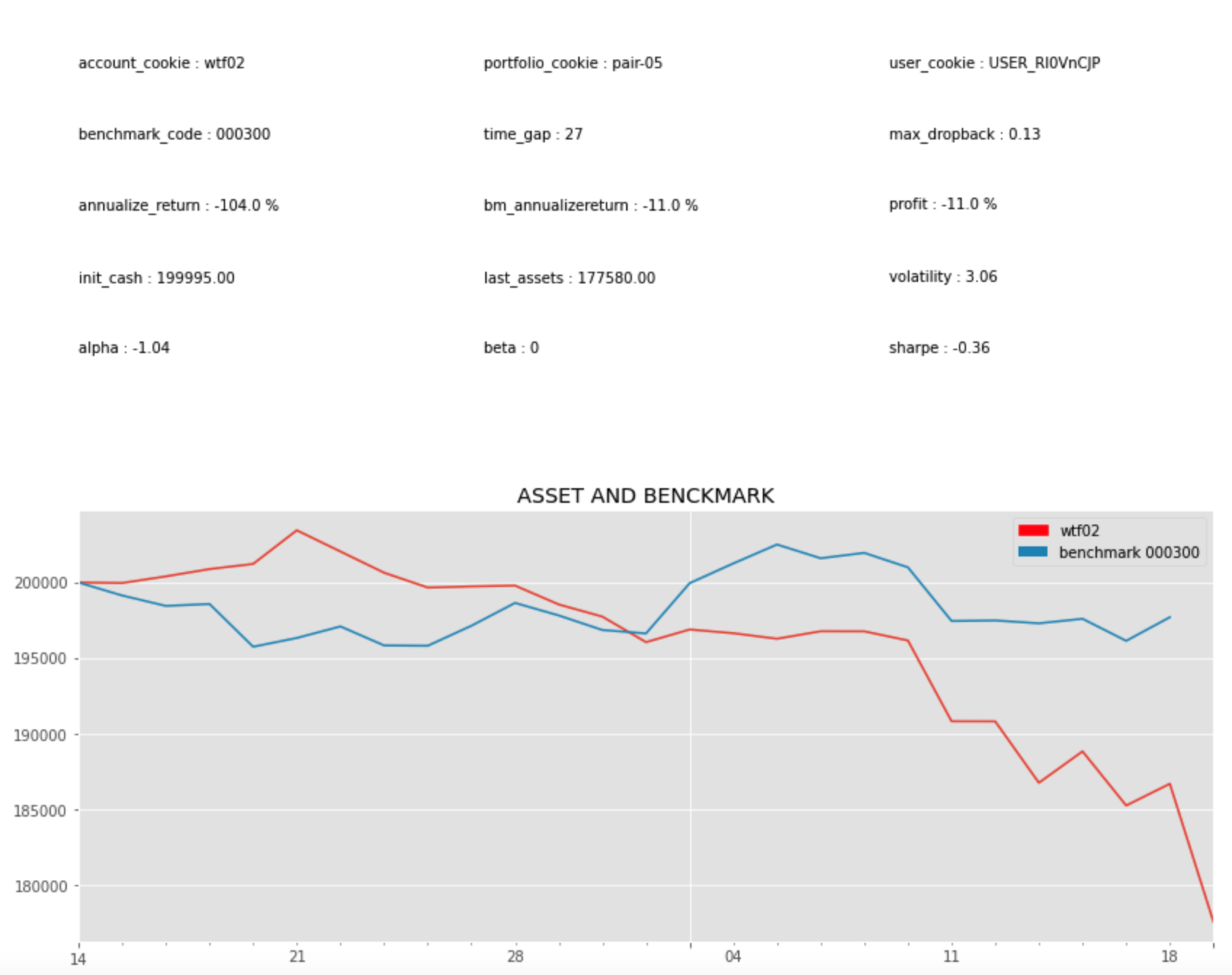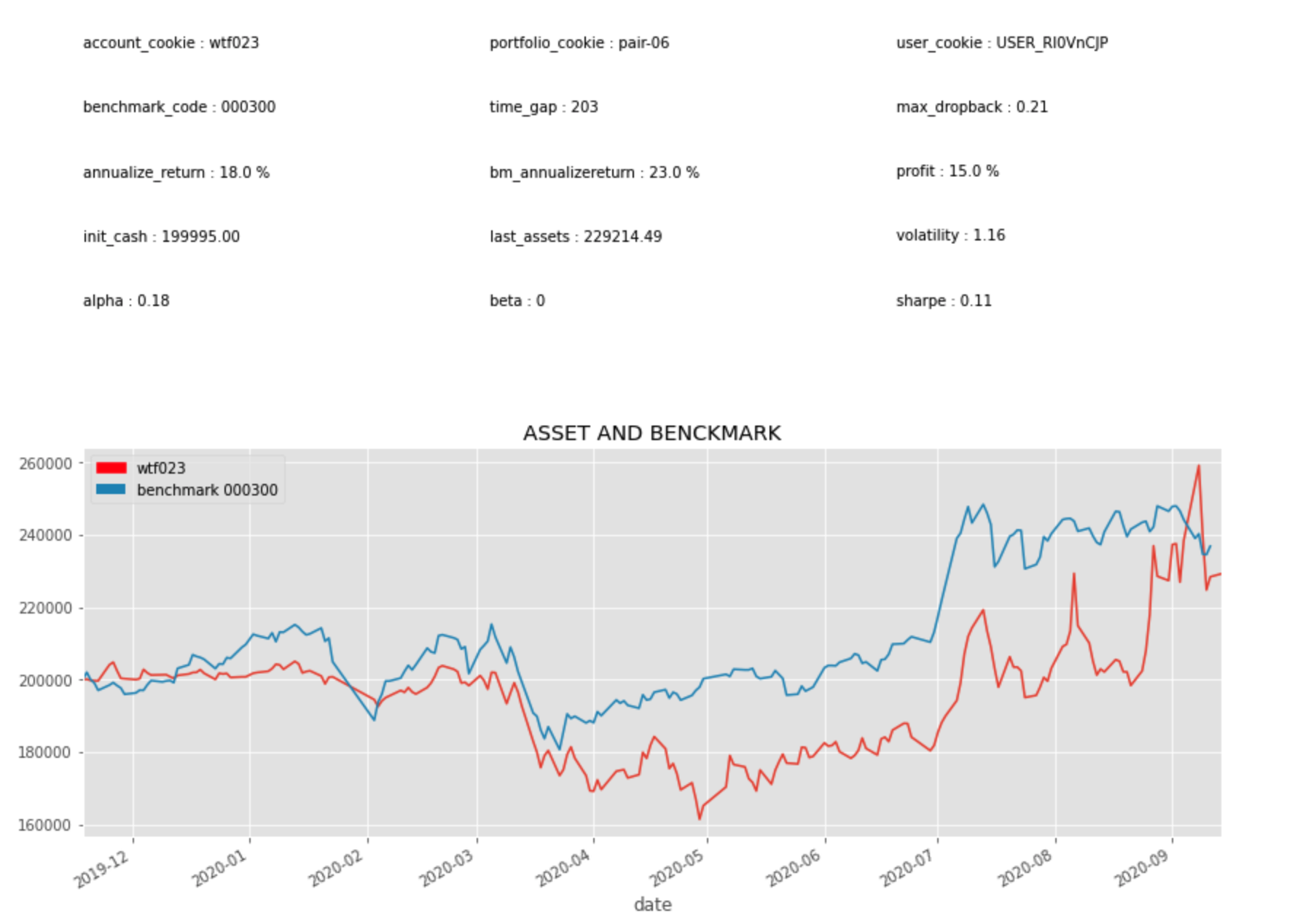## 发现配对

``````def integration_test(x, y):
result = coint(list(x), list(y))
score = result
pvalue = result
if pvalue < 0.05:
return True
return False
``````

``````def find_pairs(code_list, start_date, end_date, method_type):
n = len(code_list)
pairs = []
for i in range(n):
for j in range(i+1, n):
c1 = code_list[i]
c2 = code_list[j]
if len(S1)!= len(S2):
continue
if methods[method_type](S1, S2):
pairs.append((c1, c2))
return pairs
``````

`code_list= QA.QA_fetch_stock_block_adv().get_block('新能源').code`

``````[('002805', '300484'),
('002805', '600847'),
('002805', '300037'),
('002805', '600884'),
('600847', '300037'),
('600847', '300450'),
('600847', '600884'),
('002407', '600884')]
````````````def get_ratios(code_x, code_y):
x.index = y.index
return x.div(y, axis = 'index')
``````
``````def plot_zscore(series):
zscores = (series - series.mean()) / np.std(series)
zscores.plot()
plt.axhline(zscores.mean())
plt.axhline(1.0, color='red')
plt.axhline(-1.0, color='green')
plt.show()
``````## 执行交易

``````def pairs_backtest(code1, code2):
for i,items in enumerate(DATA.panel_gen):
item1 = items.select_code(code1)
item2 = items.select_code(code2)
order_time = items.date
if ratios[i] > 1:
if AC.sell_available.get(item1.code, 0)>0:
place_order(code1,order_time, 1000, QA.ORDER_DIRECTION.SELL,item1)
if ratios[i] < -1:
if AC.sell_available.get(item2.code, 0)>0:
place_order(code2,order_time, 1000, QA.ORDER_DIRECTION.SELL,item2)
AC.settle()
AC.save()
````````````def calc_zscore(ratios, window1, window2):
ma1 = ratios.rolling(window=window1,
center=False).mean()
ma2 = ratios.rolling(window=window2,
center=False).mean()
std = ratios.rolling(window=window2,
center=False).std()
zscore = (ma1 - ma2)/std
return zscore
``````## 写在最后

• 除去Engle-Granger方法的其他配对相关性的统计计算指标
• 应用Kalman-filter 方法判断配对交易的趋势入场点
• 交易下单的资金仓位分配策略（本文简化为每次相同的1000元）
• 其他应用最新的机器学习和深度学习方法在处理高频数据发掘配对以及判断交易入场点